# 19  Themes

Themes let you customise the non-data elements of a plot. You can change the whole theme, or update just a few elements. Here is a plot with the default theme.

``````library(ggplot2)
p1 <- ggplot(penguins, aes(x = body_mass_g,
y = bill_length_mm,
colour = species)) +
geom_point()
p1``````

## 19.1 Complete themes

You can change the whole theme to a new pre-built theme by adding one of the `theme_*()` functions.

``````p1 +
theme_classic()``````

There are many pre-built themes, some in `ggplot2`, others in the `ggthemes`, `cowplot`, and other packages. You can also write your own.

### 19.1.1 Setting the font

All the `theme_*()` functions have an argument to set the base size of the font. The default (about size 11 for most themes) is normally fine for manuscripts and theses, but too small for presentations. Increase it to 18 or 20 so the audience at the back of the auditorium can read the text.

``````p1 +
theme_classic(base_size = 18)``````

## 19.2 Setting the default theme

If you are making several figures and want them all to have the same theme, you can set the default with `theme_set()`. I do this in the first chunk of quarto and R markdown documents.

``theme_set(theme_classic())``

## 19.3 Changing individual elements of the theme

Every non-data element of the plot can be changed with `theme`. Below are a few examples.

### 19.3.1 Rotating axis labels

Sometimes long axis labels need rotating to stop them overlapping.

``````p2 <- ggplot(penguins, aes(x = species, y = body_mass_g)) +
geom_boxplot()
p2``````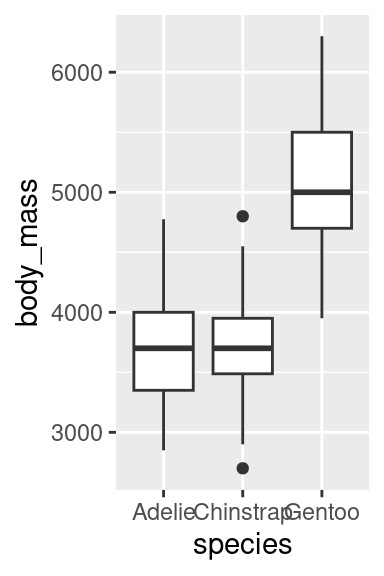``````p2 +
theme(axis.text.x = element_text(angle = 45, hjust = 1))``````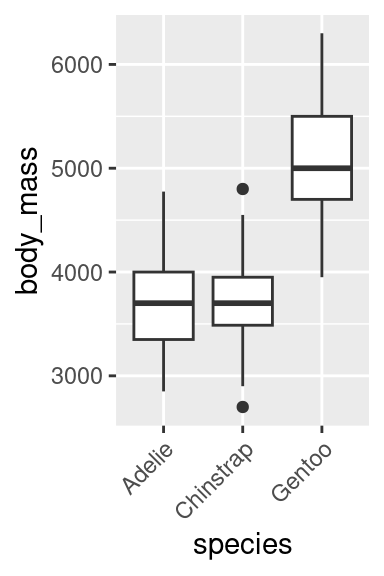Reading angled text is hard work, so consider alternative solutions to this problem, such as dodging the axis labels

``p2 + scale_x_discrete(guide = guide_axis(n.dodge=2))``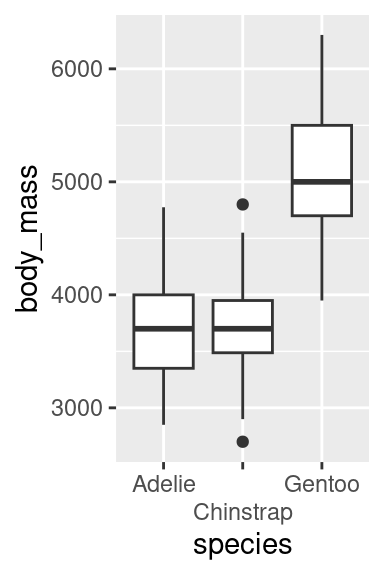Or flipping the plot.

``p2 + coord_flip()``### 19.3.2 Changing the legend position

By default, the legend is put to the right of the plot. It can be moved with the `legend.position` argument to `theme()`.

``p1 + theme(legend.position = "bottom")``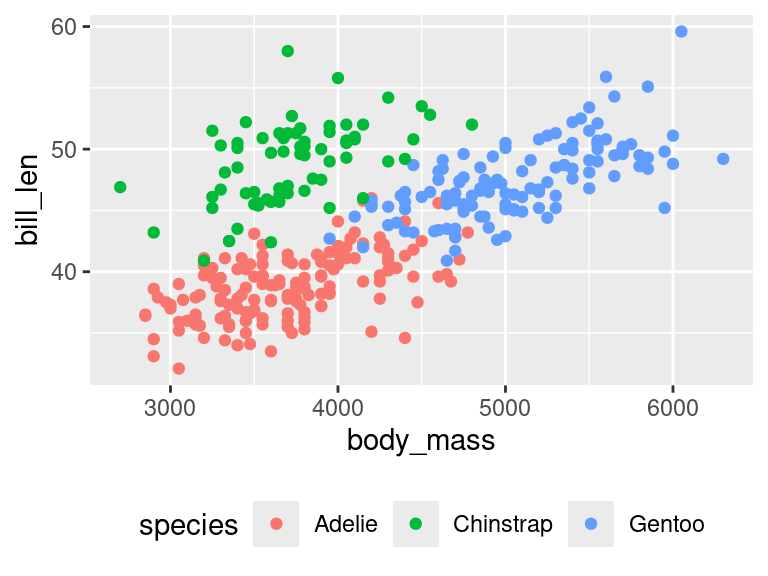If there is space, the legend can be moved into the plot by giving `legend.position` the x and y coordinates relative to the plot (0 is left or bottom, 1 is top or right). Use `legend.justification` to specify which corner of the legend should be in the position set by `legend.position` justify the legend.

``````p1 +
theme(
legend.position = c(x = 0.99, y = 0.01),
legend.justification = c(x = "right", y = "bottom"))``````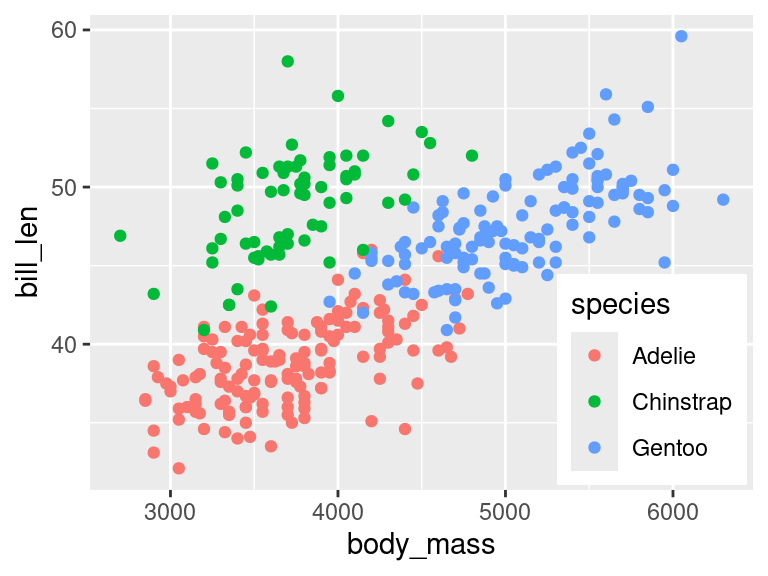The legend can be removed with

``p1 + theme(legend.position = "none")``This will remove all legends. If a plot has multiple legends, and you want to keep some of them, you get more control by using the `show.legend` argument to any of the geoms or by using `guides()`

### 19.3.3 Removing an element

You can remove non-data elements of the plot by setting them to `element_blank()`.

``p1 + theme(panel.grid = element_blank())``